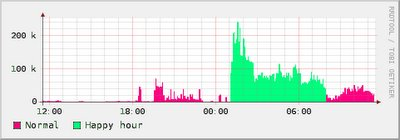## 23 February 2006

### Using rrdtool to monitor traffic on different schedules

I upgraded my broadband connection to one where there is no traffic limit during the night (appropriately referred by my ISP as "happy hours"!). I was already using rrdtool to monitor the total incoming traffic of my router, but now I wanted to separate the traffic according to a schedule.

It was obvious that at least two data sources are needed: one for the normal (limited) traffic and another for the happy (unlimited) traffic. The first (naive) approach was to create these two data sources plus an automatically computed total data source, as

```rrdtool create \$RRD \
-s \$STEP \
DS:normal:COUNTER:\$HEARTBEAT:\$MIN:\$MAX \
DS:happy:COUNTER:\$HEARTBEAT:\$MIN:\$MAX \
DS:total:COMPUTE:normal,happy,+ \
RRA:AVERAGE:\$XFF:\$((\$STEP/\$STEP)):\$((2*\$DAY/\$STEP)) \
RRA:AVERAGE:\$XFF:\$((\$HOUR/\$STEP)):\$((2*\$MONTH/\$HOUR)) \
RRA:AVERAGE:\$XFF:\$((\$DAY/\$STEP)):\$((2*\$YEAR/\$DAY))
```

and feed either according to the current time of the day:

```TIME=N
COUNTER=`snmpget ...`

TIMEOFDAY=`date +'%H%M'`
if [ \$TIMEOFDAY -gt \$HAPPYSTART -a \$TIMEOFDAY -le \$HAPPYEND ]
then
NORMAL=0
HAPPY=\$COUNTER
else
NORMAL=\$COUNTER
HAPPY=0
fi

rrdtool update \$RRD \$TIME:\$NORMAL:\$HAPPY
```

where HAPPYSTART and TIMEOFDAY have the happy hour start and end times in hhmm format.

However this does not work as expected due to the way rrdtool treats the COUNTER data sources, and the impact of the schedule transition on such treatment. For illustration purposes, suppose in one instant t1 the counter value is 1000 bytes and in the normal schedule, and the next instant t2 the counter value is 1001 bytes but on the happy schedule. The updates will be

```t1:1000:0
t2:0:1001
```

but rrdtool will see and count the happy counter vary from 0 to 1001, therefore counting 1001 bytes, and not the correct value of 1 byte!

The solution is have only one COUNTER, and separate the traffic kind using a factor in the [0, 1] range as:

```rrdtool create \$RRD \
-s \$STEP \
DS:total:COUNTER:\$HEARTBEAT:\$MIN:\$MAX \
DS:ratio:GAUGE:\$HEARTBEAT:0:1 \
DS:normal:COMPUTE:total,ratio,* \
DS:happy:COMPUTE:total,1,ratio,-,* \
RRA:AVERAGE:\$XFF:\$((\$STEP/\$STEP)):\$((2*\$DAY/\$STEP)) \
RRA:AVERAGE:\$XFF:\$((\$HOUR/\$STEP)):\$((2*\$MONTH/\$HOUR)) \
RRA:AVERAGE:\$XFF:\$((\$DAY/\$STEP)):\$((2*\$YEAR/\$DAY))
```

and for the update:

```TIME=N
COUNTER=`snmpget ...`

TIMEOFDAY=`date +'%H%M'`
if [ \$TIMEOFDAY -gt \$HAPPYSTART -a \$TIMEOFDAY -le \$HAPPYEND ]
then
RATIO=0
else
RATIO=1
fi

rrdtool update \$RRD \$TIME:\$COUNTER:\$RATIO
```

With this I get nice pictures such as this one: